Browse by Topic
Related Topics

# Analyzing Pedometer Data with Mathematica

In Stephen Wolfram’s personal analytics blog post, he showed a number of interesting plots of the steps he’s recorded on his pedometer over the past two years. Each plot highlighted a different feature of his activity. For example, this daily step distribution makes it clear that Stephen is typically most physically active around noon: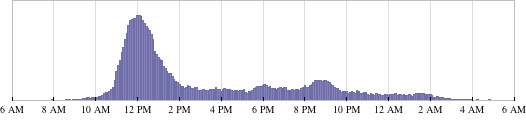In this blog post I’ll show you how to analyze your own pedometer data and make cool plots like Stephen’s. If you don’t have any data, you can use the attached sample data that corresponds to my own physical activity.

First we need to import the data and format it appropriately.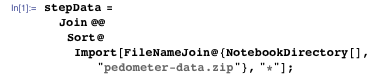The data is formatted as pairs of time stamps and step counts in five-minute intervals.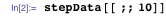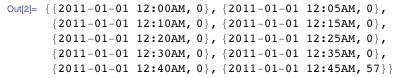We need to get the time stamps into a Mathematica-friendly format. We’ll use DateList, which converts a date string to a numeric representation of the date: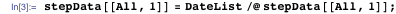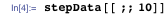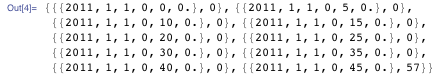To make a daily distribution like the one shown above, we’ll need to find the total number of steps in each five-minute time interval. We’ll start by creating a list of all the start times of the five-minute intervals in a day, represented by hour-minute pairs: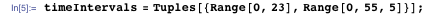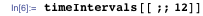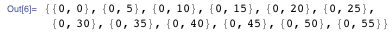Now we’ll define a function that takes an interval start time and returns the number of steps taken in that interval. We’ll give it a default value of zero: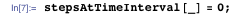Next, we’ll iterate through our data and add the number of steps taken at each time stamp to the value of our function associated with that time: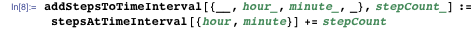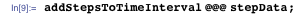Given a time interval, our function will now return the number of steps taken in that interval. For example, we can check how many steps I took in the five-minute interval that starts at noon over all the days recorded: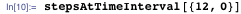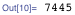We can apply the stepsAtTimeInterval function to all the time interval values to get data suitable for a distribution plot: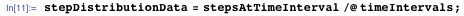Finally, we’ll rotate the data so that our plot starts at 6am rather than midnight: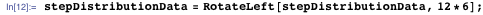Now let’s plot it: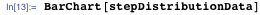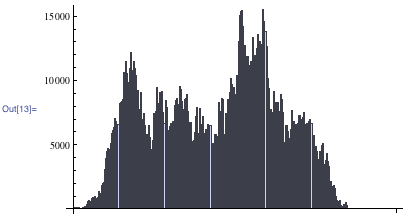Making styling adjustments is easy. We just give BarChart a few options. Here’s the same plot with styling tweaks to make it look just like Stephen’s: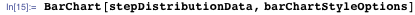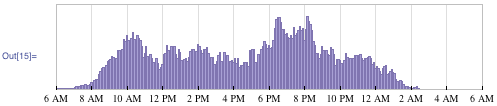And now to make the diurnal plot, we’ll start by taking just the step counts from our data: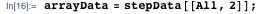Next, we’ll partition the data so that the steps for each day are grouped together. There were five minutes between entries, so each day will have 12 entries for each hour in the day: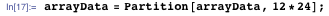Finally, we need to reverse the entries in each day and transpose the whole set of data so that it’s in the right orientation for ArrayPlot: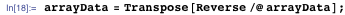Now we can plot it: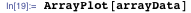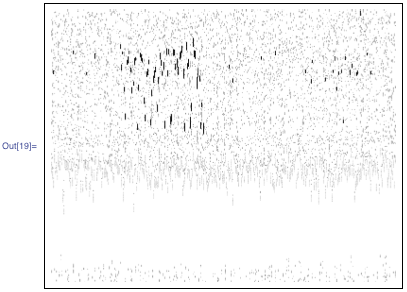In this plot, each five-minute interval is represented by a rectangle that is colored based on the number of steps I took in that interval. The time of day is plotted along the y axis and the date is plotted along the x axis.

Again, we can make some tweaks to get our plot looking just like Stephen’s: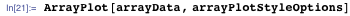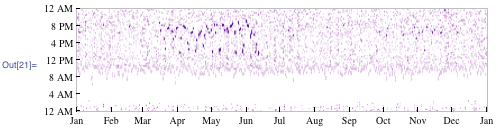The cluster of dark streaks from mid-March to mid-June corresponds to when I was training for a 10k race. They show up as peaks around 6pm and 8pm in the daily distribution we plotted above. This makes sense, since I typically run after work. There are also some darker spots in October and November that correspond to my less intense training for a 5k race.

The last plot we’ll do is a stack of two time series. The top time series will be of daily step totals. The bottom time series will be of monthly averages of those totals, which will give us a broader picture of how activity levels have changed over time.

To begin with, we’ll define a couple of functions to extract the day and month respectively from a single-step data entry. We could use the Part function, but this will make things more clear: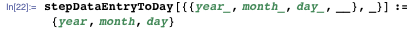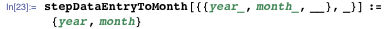Now we’ll define a function that takes a list of step data entries and returns a list of daily step totals: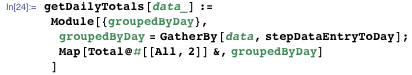It works by first gathering the entries by day, then summing the step counts in each group.

In order to plot the daily totals using DateListPlot, we need both the daily totals and the dates: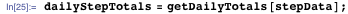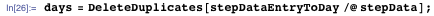Now we can transpose them to get our plot data, and plot it: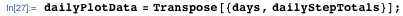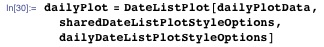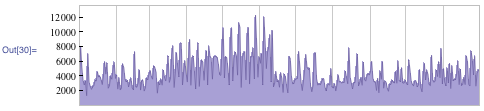As before, I’ve used styling options to make our plot match Stephen’s.

Next we want to get the monthly averages of the daily totals. We’ll start by grouping the entries by month: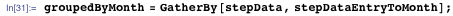Now we can use the function we defined for getting daily totals on the groups of entries and calculate the mean of those totals: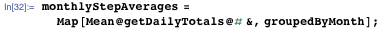Finally, we’ll get the list of months and transpose that with the averages to get our plot data: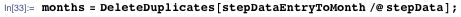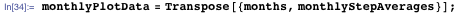Let’s plot it: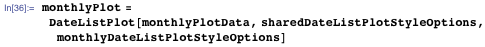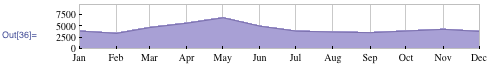And now we can stack them using Column: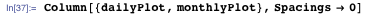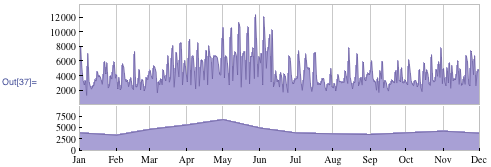The daily time series shows a clear increase in steps leading up to my 10k. My activity levels drop off after the race in mid-June. We can also see a gradual incline in the monthly time series that peaks in November. This corresponds to my training for the 5k I ran on Thanksgiving.

Well that was fun! We were able to use Mathematica to import, format, and plot our pedometer data. Analyzing our data is a great way to learn about our own habits and hopefully make improvements based on those insights. It looks like I need to find another race to motivate me to stay active!

Download this ZIP file that includes the post as a CDF file, associated data files, and the code used above.